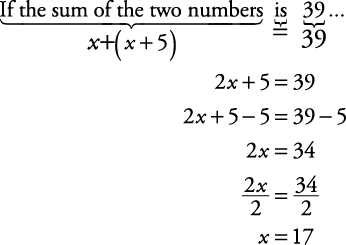## Number Problems

Here are some examples solving number problems.

##### Example 1

When 6 times a number is increased by 4, the result is 40. Find the number.

First, circle what you must find— the number. Letting x stand for the number gives the equation

6 x + 4 = 40

Subtracting 4 from each side gives

6 x = 36

Dividing by 6 gives

x = 6

So the number is 6.

##### Example 2

One number exceeds another number by 5. If the sum of the two numbers is 39, find the smaller number.

First, circle what you are looking for— the smaller number. Now, let the smaller number equal x. Therefore, the larger number equals x + 5. Now, use the problem to set up an equation.Therefore, the smaller number is 17.

##### Example 3

If one number is three times as large as another number and the smaller number is increased by 19, the result is 6 less than twice the larger number. What is the larger number?

First, circle what you must find— the larger number. Let the smaller number equal x. Therefore, the larger number will be 3 x. Now, using the problem, set up an equation.Therefore, the larger number, 3 x, is 3(5), or 15.

##### Example 4

The sum of three consecutive integers is 306. What is the largest integer?

First, circle what you must find— the largest integer. Let the smallest integer equal x; let x + 1 equal the next integer; let the largest integer equal x + 2. Now, use the problem to set up an equation.Therefore, the largest integer, x + 2 = 101 + 2 = 103.